# Lions Weekly: November 10, 2021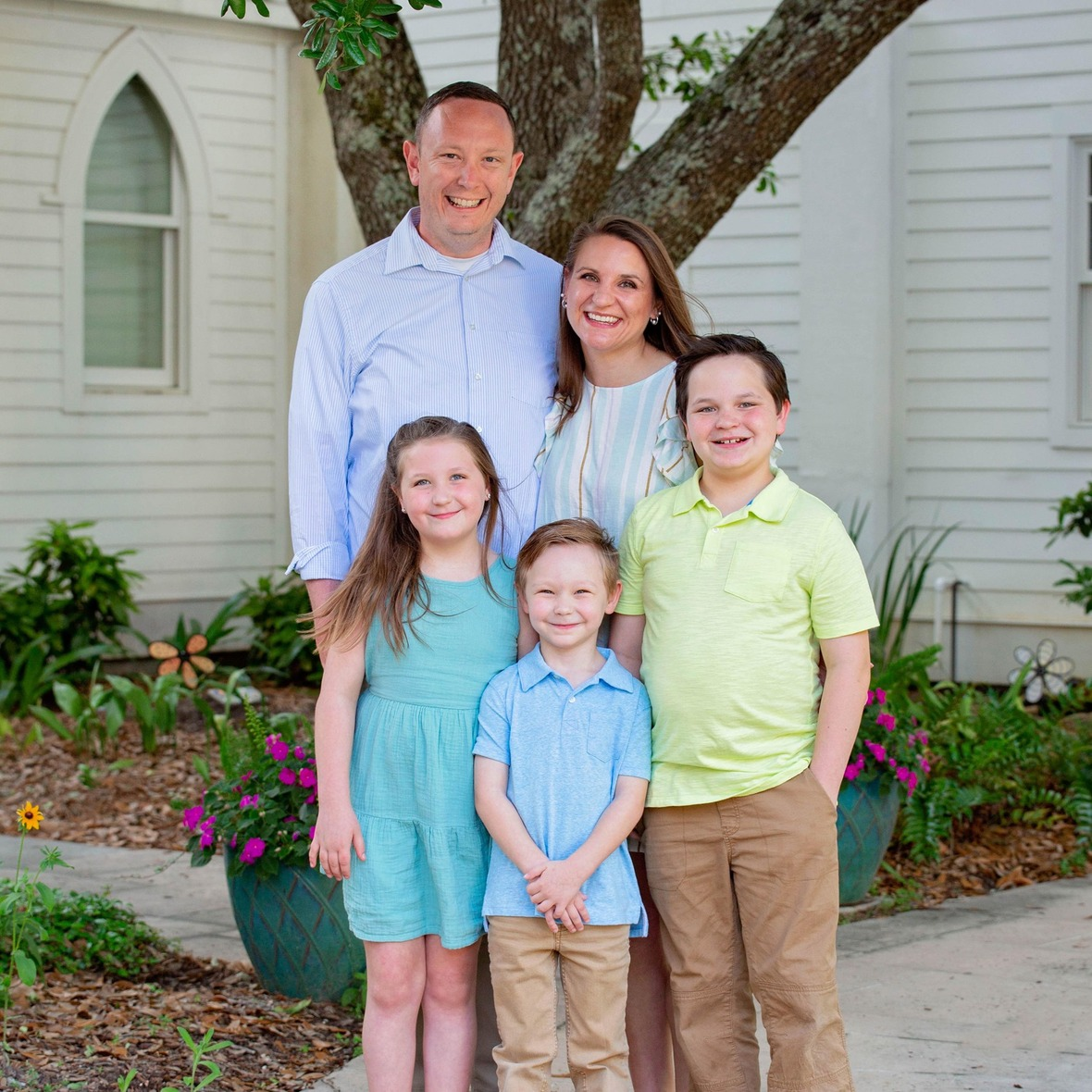# A Note From Mr. Sandefur

Lion Family,

I genuinely look forward to my opportunity weekly to share with each of you as we partner together to develop servant leaders who will change the world for Christ. When I last addressed you, I did so from the ACSI Flourishing Schools Institute. Along with our administrators who participated, I was blessed to attend and learn from leading thinkers from across the nation and colleagues who actively serve in faith-based education daily. Through my conversations, I became humbled by the challenges that we face in today’s society as we not only seek to educate students but seek to do so in a loving, faith-based environment. It became more evident than ever that our students continue to be exposed to issues that you and I did not face when we were growing up, and the impact of such challenges on their learning can be detrimental.

Through this process, our leadership team has been convicted on ways to continue to partner with families to offer an excellent Christ-centered education as we seek to link arms with each of you. I look forward to sharing some of the initiatives, strategies, and changes that will become a part of our school community in the days and months ahead.

I also want to take the opportunity to congratulate our TKA football team on their playoff victory on Friday. Our team will face off against the number one team in the state in Jackson, TN, this Friday. Would you please join me in cheering for them as they prepare? Finally, I would like to encourage you to attend our upcoming choral Christmas production, as I know that you will be truly blessed as we celebrate the birth of Christ.

In Christ Alone,

Jeremy Sandefur
President

 table div table+table+table+table div table{width:100%;padding:0}table div table+table+table+table div table img{width:96.23%;padding:0;float:none}table div table+table+table+table div table td{width:100%;padding:0 1.88% 18px}/* styles */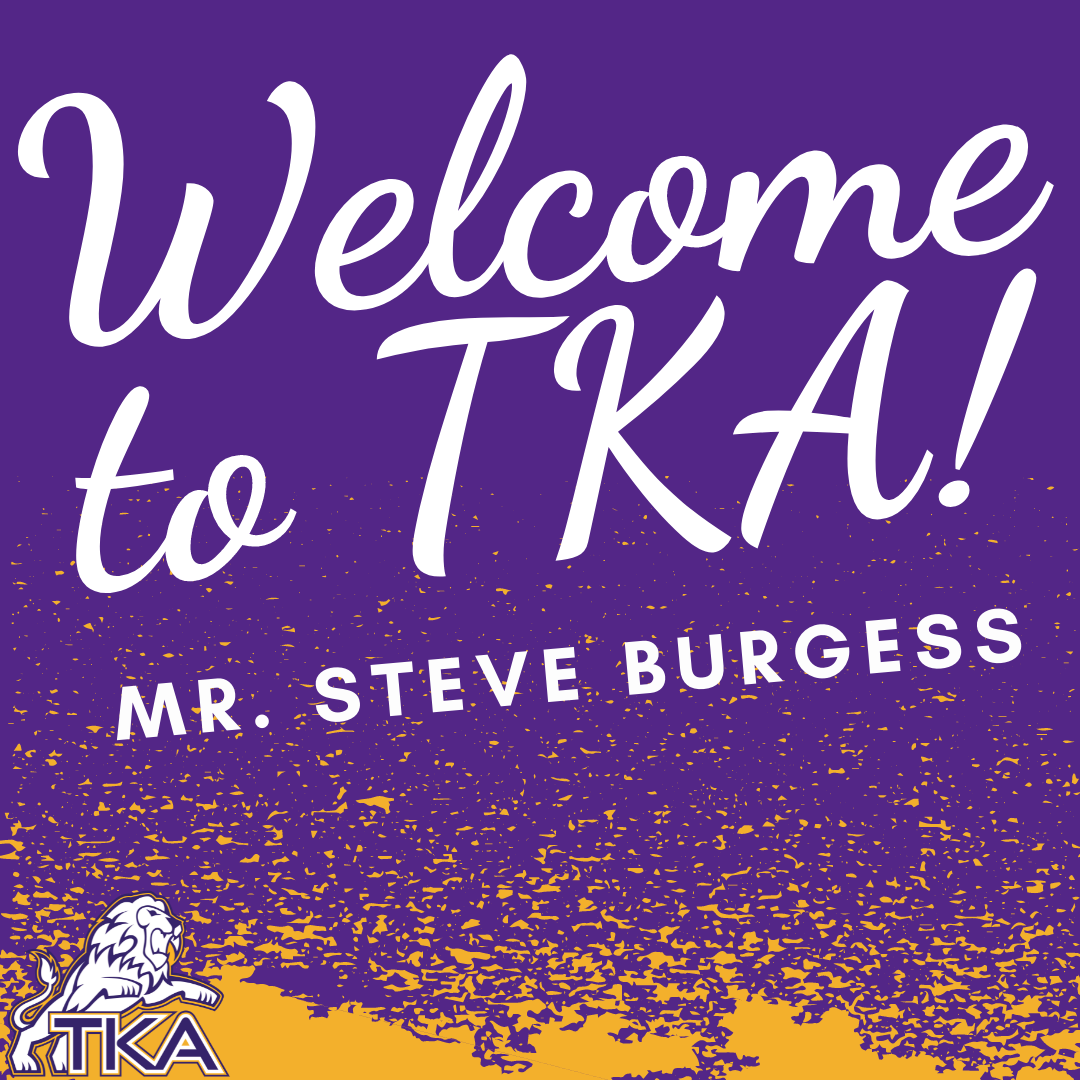# Middle School Bible

We are pleased to announce Mr. Steve Burgess as our new Middle School Bible teacher. After many weeks of prayer and deliberation, we found peace in the decision to move forward with Mr. Burgess. He brings 25+ years of public and private classroom experience to TKA in addition to 40+ years as a bi-vocational minister. Mr. Burgess earned his Master's Degree in Administration from the University of Alabama and a Master's of divinity from New Orleans Baptist Theological Seminary. He is also the current pastor of Kinzel Springs Baptist Church in Townsend. We are incredibly blessed to have Mr. Steve Burgess partner with us at TKA.

 table div table+table+table+table+table+table div table{width:100%;padding:0}table div table+table+table+table+table+table div table img{width:96.23%;padding:0;float:none}table div table+table+table+table+table+table div table td{width:100%;padding:0 1.88% 18px}/* styles */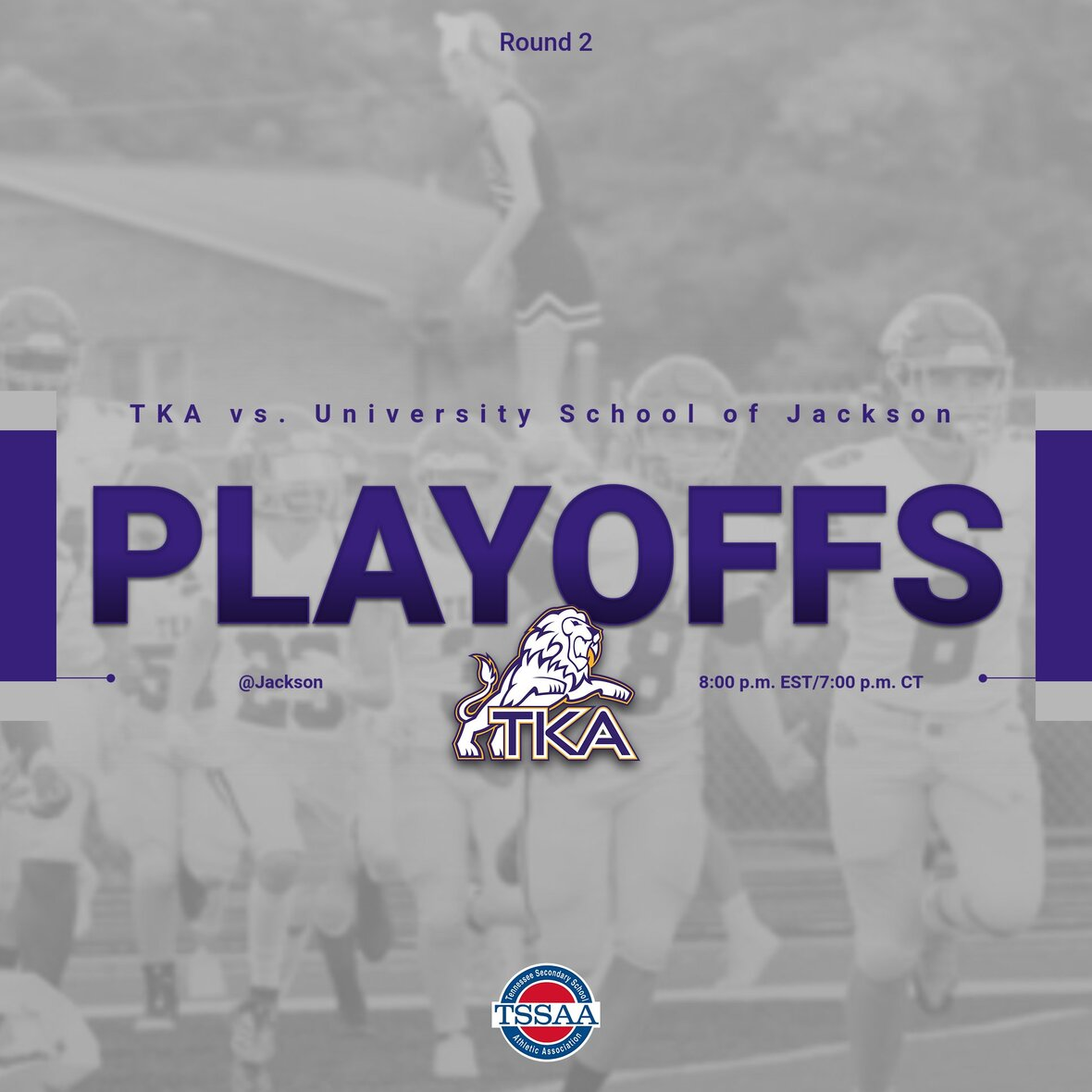# Varsity Football

Congratulations to our Lions on their win over Grace Christian of Franklin on Friday!

This Friday night, TKA will continue in the second round of the TSSAA Division II-A Football against the University School of Jackson.

Because this is a TSSAA Playoff game, a few things will be slightly different than a typical Friday night at TKA. ALL tickets will be sold via the GoFan app and are \$8. Tickets will NOT be sold at the gate. There will be no free admission or passes honored at the gate (except for players, cheerleaders, and band members). The only passes that will be honored are TSSAA ID Cards. Lastly, please note that kickoff is set for 8:00 p.m.EST/7:00 p.m. CT.

To purchase tickets for Friday's round 2 playoff game vs. University School of Jackson, please click here.

 /* styles */
 table div table+table+table+table+table+table+table+table+table div table{width:100%;padding:0}table div table+table+table+table+table+table+table+table+table div table img{width:96.23%;padding:0;float:none}table div table+table+table+table+table+table+table+table+table div table td{width:100%;padding:0 1.88% 18px}/* styles */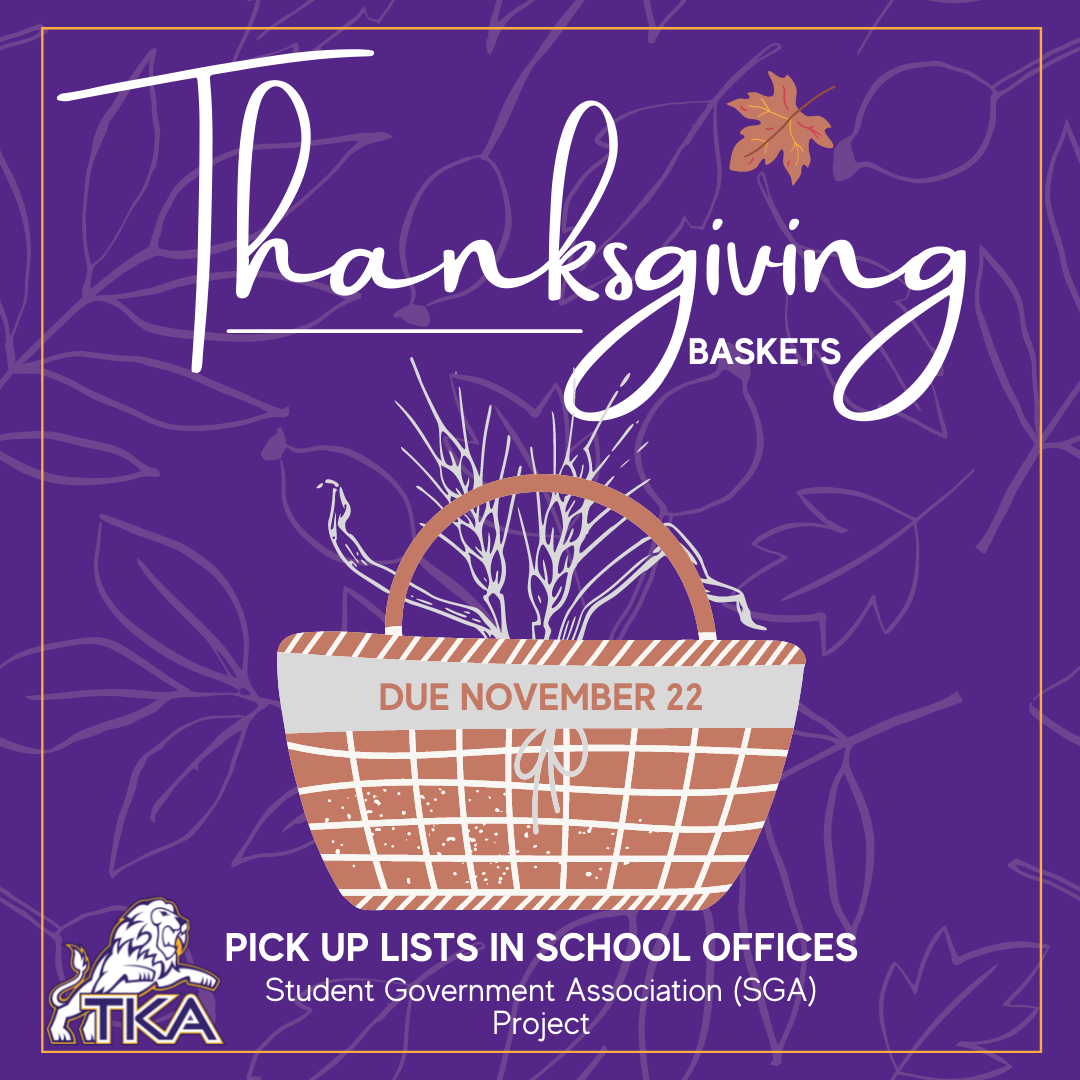SGA is partnering with our TKA families to distribute Thanksgiving baskets for those in need. If you would like to help, pick up a basket in the school offices, fill it with the listed items, or click here to download the list of needed items. Items and baskets need to be returned by Monday the 22nd. When returning items from the list, please be sure the items are returned in a basket or placed in a basket located in one of the school offices.

 table div table+table+table+table+table+table+table+table+table+table+table div table{width:100%;padding:0}table div table+table+table+table+table+table+table+table+table+table+table div table img{width:96.23%;padding:0;float:none}table div table+table+table+table+table+table+table+table+table+table+table div table td{width:100%;padding:0 1.88% 18px}/* styles */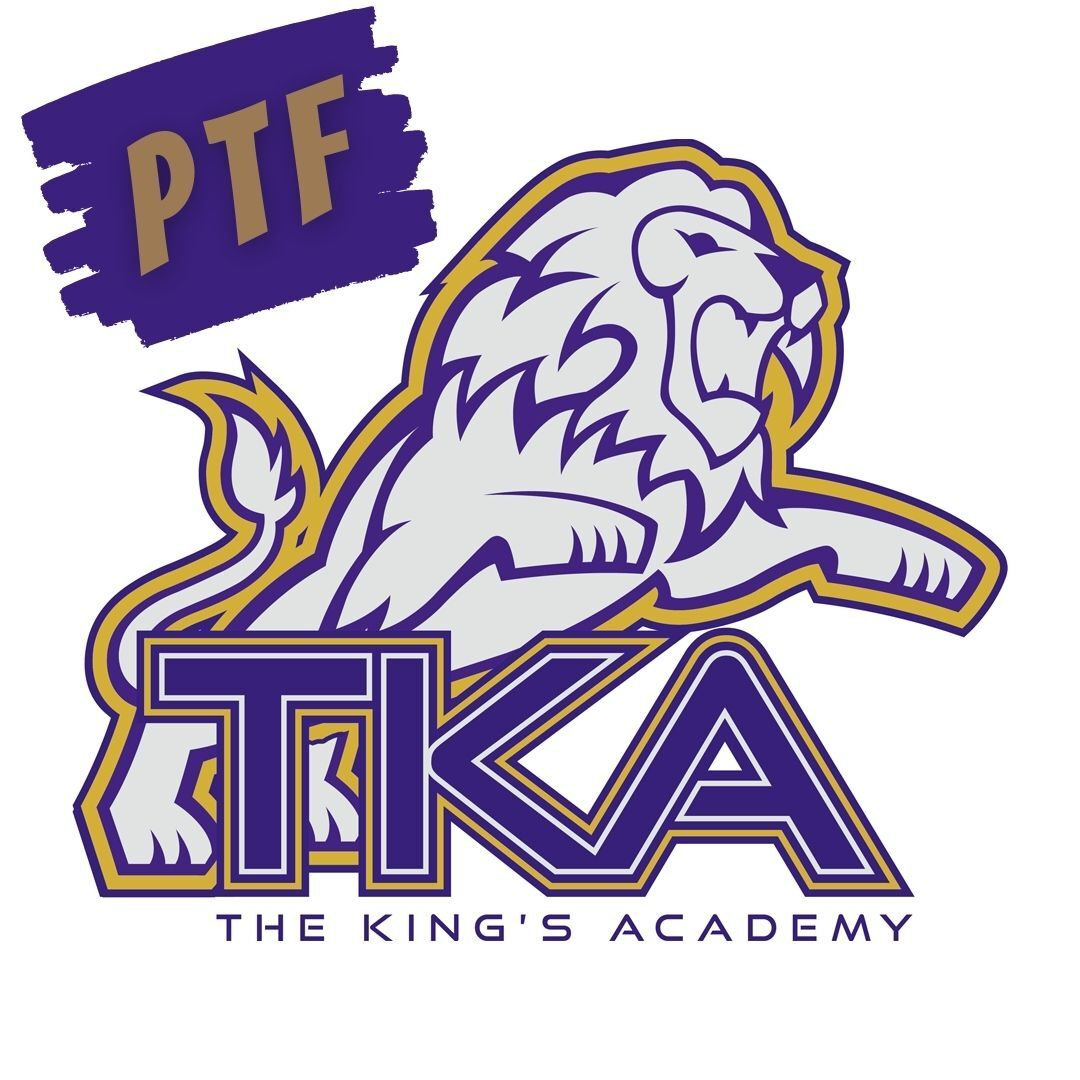# PTF

The next PTF meeting will be on Thursday, November 18th, from 3:15 PM-4:05 PM in Brandes. Childcare will be provided throughout the aftercare program free of charge until 4:15 PM for the meeting.

If you haven't joined PTF, no worries, come anyway! Membership forms will be available at the meeting. The fee to join is \$25 per family.

We look forward to having you as part of our PTF this year!

 table div table+table+table+table+table+table+table+table+table+table+table+table+table div table{width:100%;padding:0}table div table+table+table+table+table+table+table+table+table+table+table+table+table div table img{width:96.23%;padding:0;float:none}table div table+table+table+table+table+table+table+table+table+table+table+table+table div table td{width:100%;padding:0 1.88% 18px}/* styles */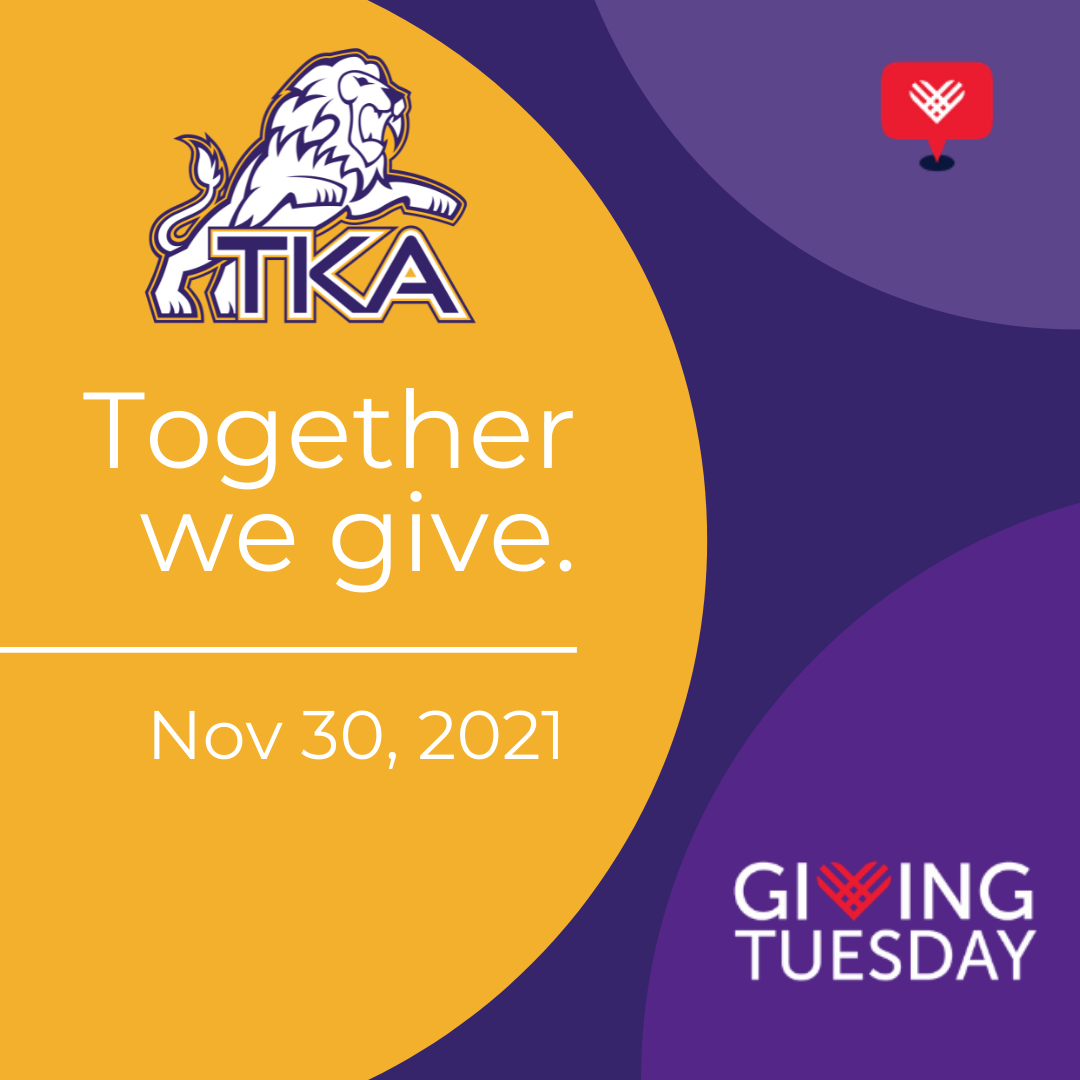# Giving Tuesday

National Giving Tuesday is a day set aside to support non-profit organizations. The King’s Academy seeks to honor our 142 years of faithful Christian education by asking for support from 142 donors on Giving Tuesday. All gifts will be incredibly beneficial as we seek to develop servant leaders for Christ. Would you consider being 1/142 by partnering with us on November 30? Donations can be made by clicking here or in the school office. Gifts will go towards helping us achieve upgrades in curriculum, facilities and student life on campus. All donations are tax-deductible.

Please consider sharing this opportunity with your family and friends as we work together for our TKA school community.

 table div table+table+table+table+table+table+table+table+table+table+table+table+table+table+table div table{width:100%;padding:0}table div table+table+table+table+table+table+table+table+table+table+table+table+table+table+table div table img{width:96.23%;padding:0;float:none}table div table+table+table+table+table+table+table+table+table+table+table+table+table+table+table div table td{width:100%;padding:0 1.88% 18px}/* styles */# TKA Choral Department

Please mark your calendars and save the dates for our upcoming Christmas performances!

▪ December 3 at 8:30 a.m. and 1:30 p.m. in Woody Auditorium.
▪ December 4 at 6:00 p.m. in Woody Auditorium.
▪ December 5 at 6:00 p.m. at Everett Hills Baptist Church.
 ▪ December 3 at 8:30 a.m. and 1:30 p.m. in Woody Auditorium.
 ▪ December 4 at 6:00 p.m. in Woody Auditorium.
 ▪ December 5 at 6:00 p.m. at Everett Hills Baptist Church.

We look forward to celebrating This is Christmas with you!

 table div table+table+table+table+table+table+table+table+table+table+table+table+table+table+table+table+table div table{width:100%;padding:0}table div table+table+table+table+table+table+table+table+table+table+table+table+table+table+table+table+table div table img{width:96.23%;padding:0;float:none}table div table+table+table+table+table+table+table+table+table+table+table+table+table+table+table+table+table div table td{width:100%;padding:0 1.88% 18px}/* styles */# Love Gala

Save the date for the first annual Love Gala hosted by The King's Academy. The Love Gala will be on February 12, 2022, at Johnson University in Knoxville, TN. Plan on joining us for a night of fellowship, an exciting auction with great items, and a time to honor our alumni, teachers, and those who have left a legacy at TKA.

Each ticket purchased for the event will have an opportunity to win FREE tuition for one student for the 2022-23 academic year. Additional tuition remission opportunity tickets are available for purchase at the door for \$25 per opportunity. All opportunity drawing tickets are Non-Transferable. Only the student drawn is eligible for tuition remission. Must be present to receive tuition remission.

 table div table+table+table+table+table+table+table+table+table+table+table+table+table+table+table+table+table+table+table div table{width:100%;padding:0}table div table+table+table+table+table+table+table+table+table+table+table+table+table+table+table+table+table+table+table div table img{width:96.23%;padding:0;float:none}table div table+table+table+table+table+table+table+table+table+table+table+table+table+table+table+table+table+table+table div table td{width:100%;padding:0 1.88% 18px}/* styles */# Empukani Primary School Update

Thank you to all who helped and donated to provide new desks for the students at Empukani Primary school in Kenya. Here are some pictures of the students with their new desks! The King’s Academy is proud to be partnering with Mission Maasai to reach students in Empukani, Kenya.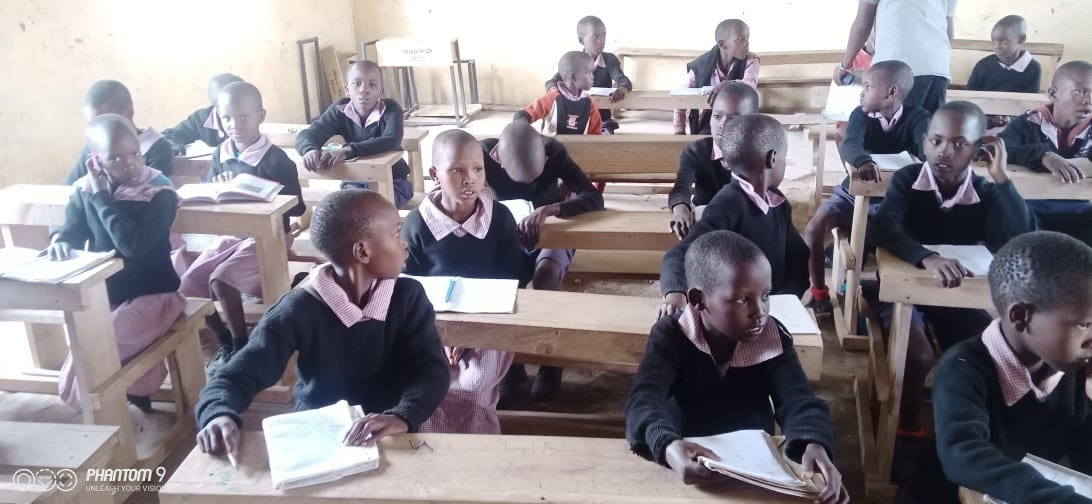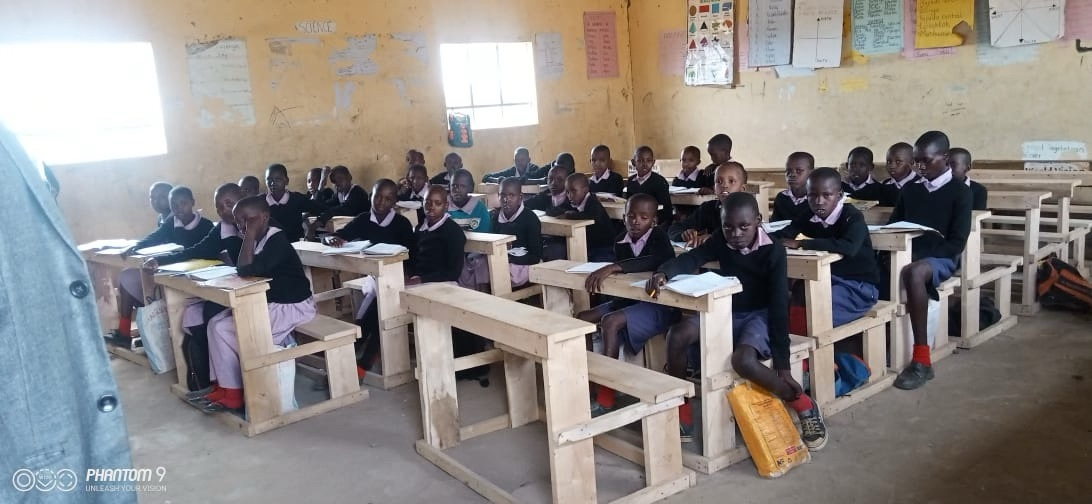table div table+table+table+table+table+table+table+table+table+table+table+table+table+table+table+table+table+table+table+table+table+table+table div table{width:100%;padding:0}table div table+table+table+table+table+table+table+table+table+table+table+table+table+table+table+table+table+table+table+table+table+table+table div table img{width:96.23%;padding:0;float:none}table div table+table+table+table+table+table+table+table+table+table+table+table+table+table+table+table+table+table+table+table+table+table+table div table td{width:100%;padding:0 1.88% 18px}/* styles */# Veterans Day

We are truly thankful for all who have fought and are currently doing so for our freedoms. Please join us as we honor those who have served faithfully this Veterans Day.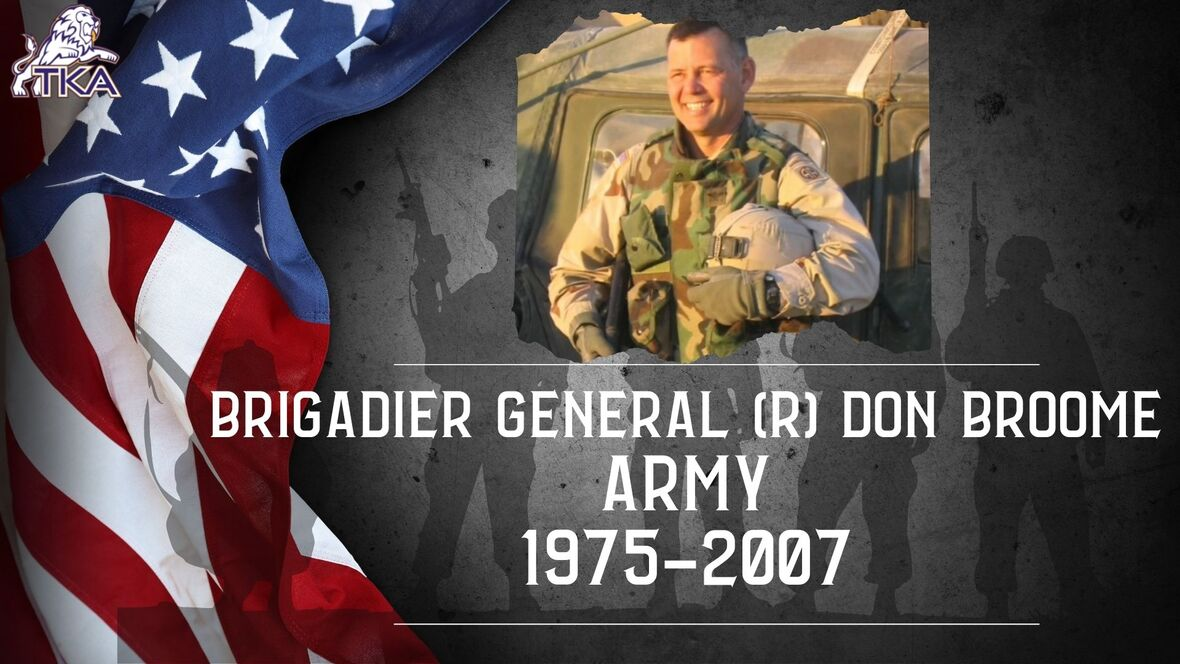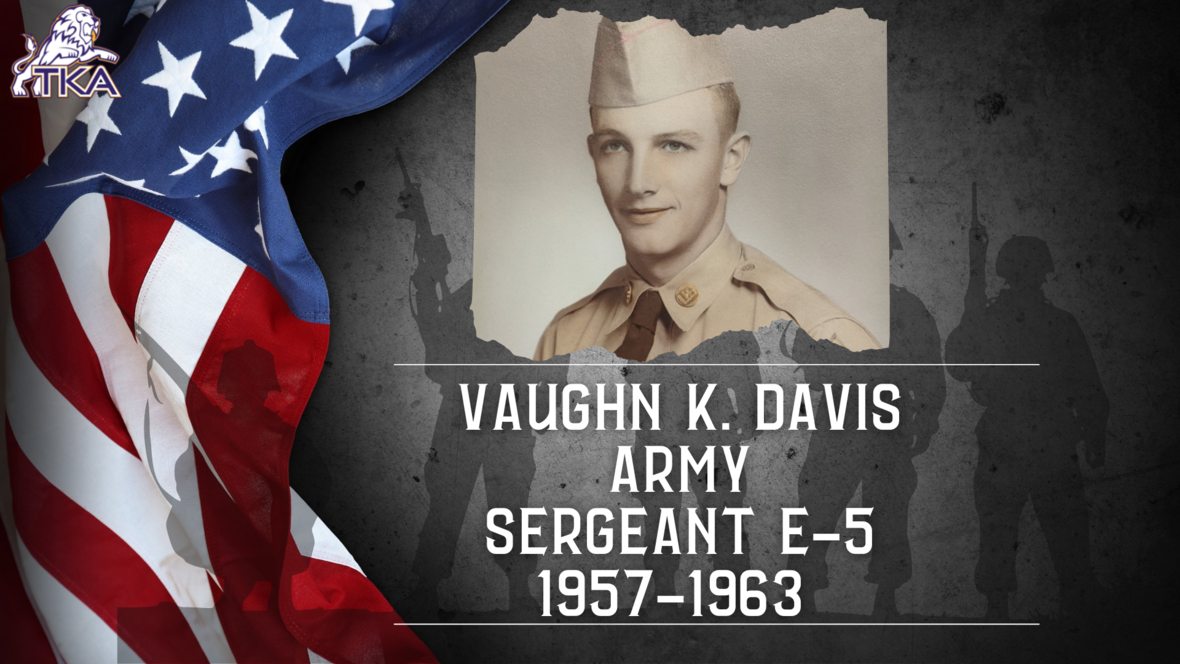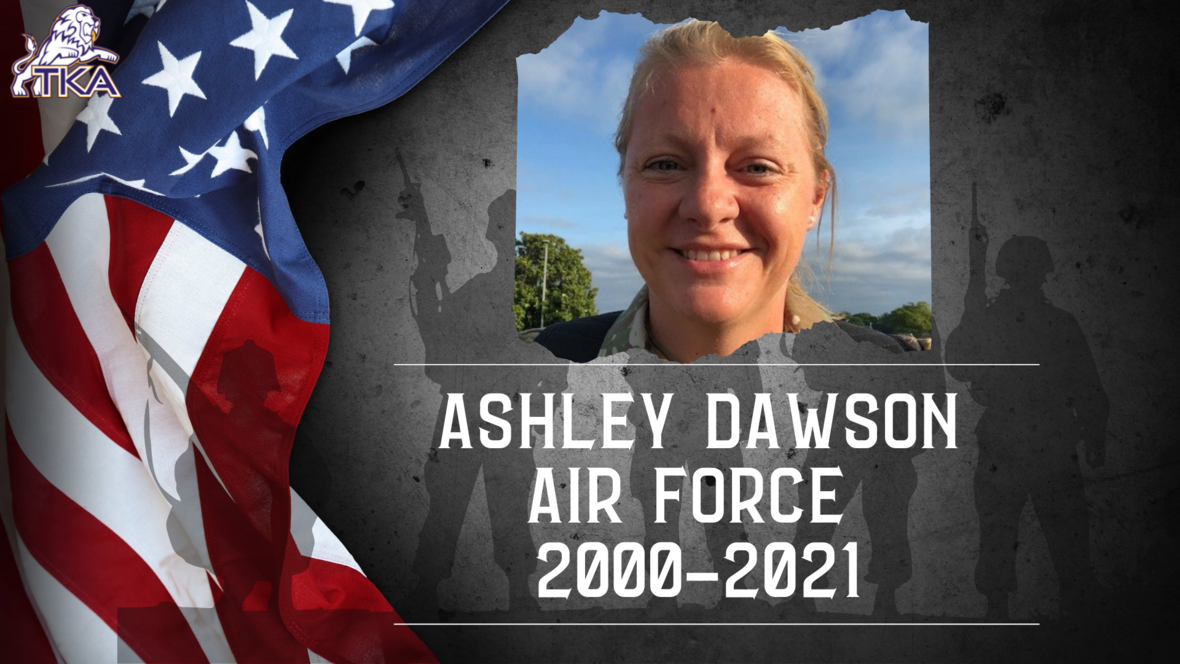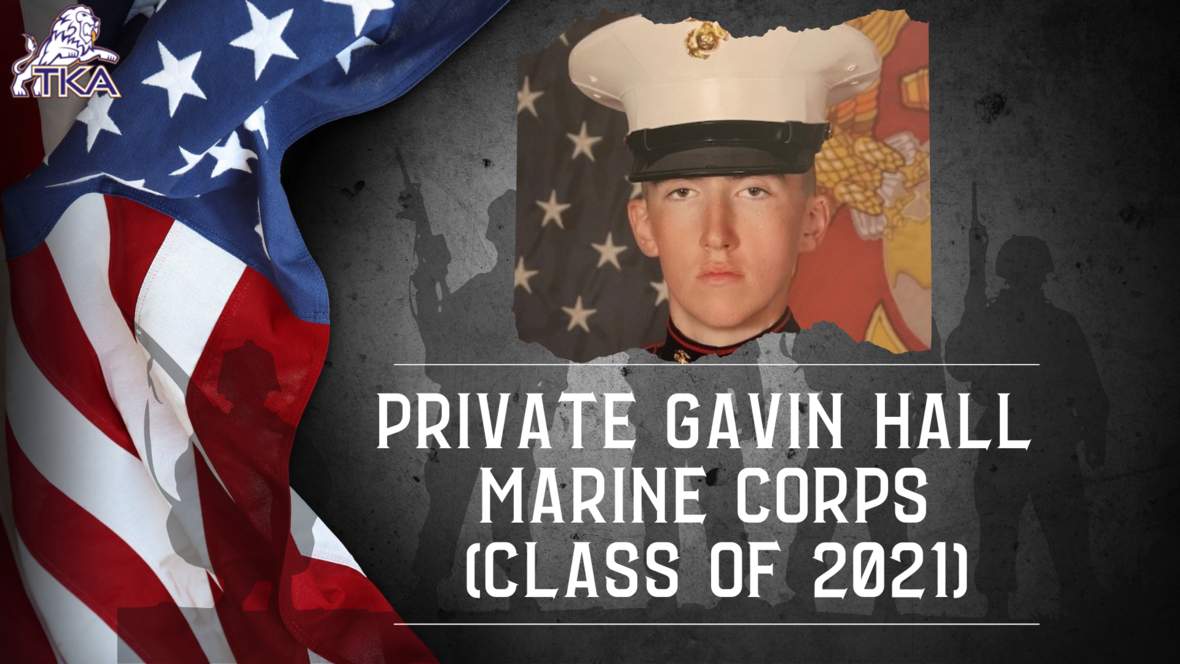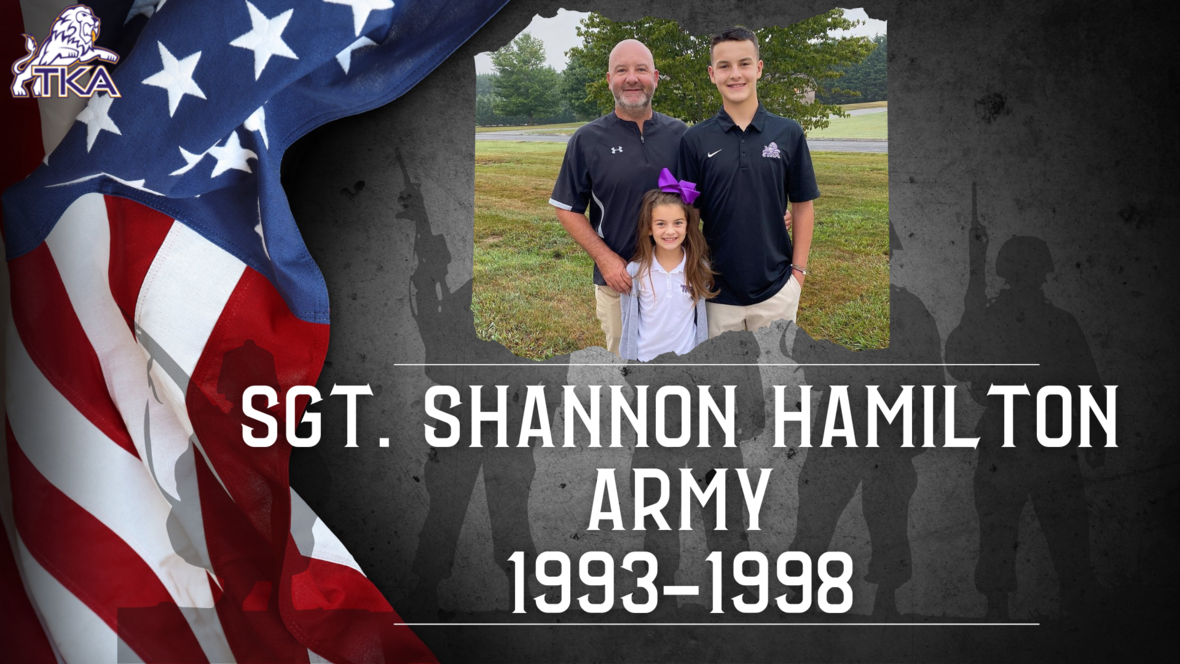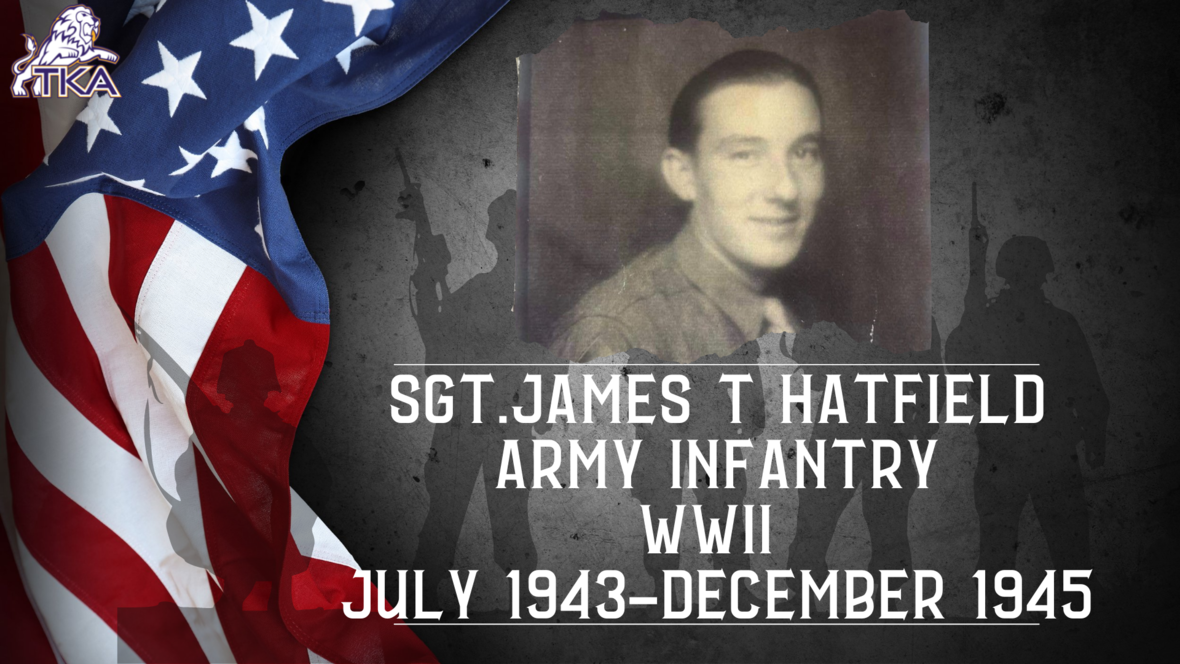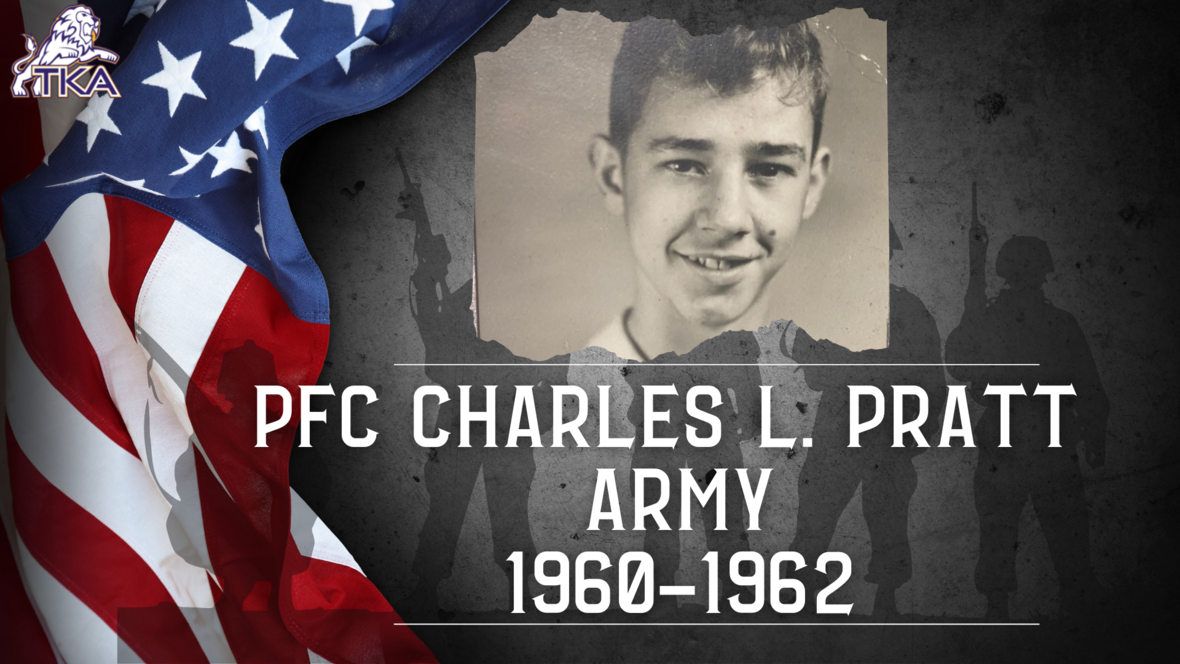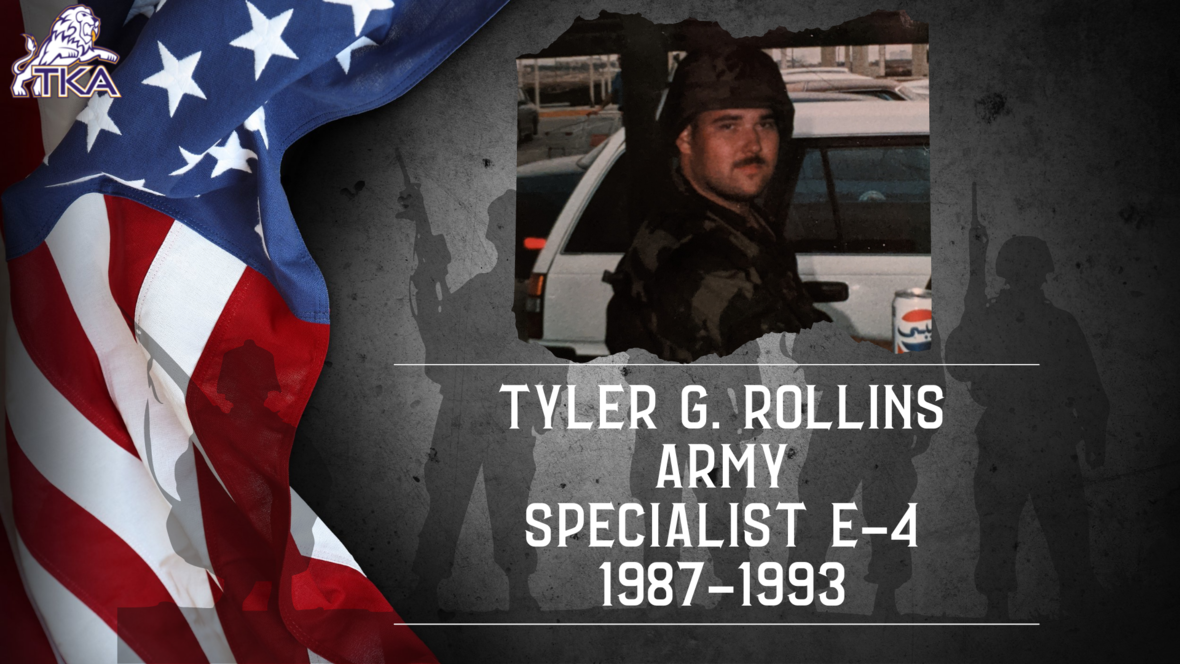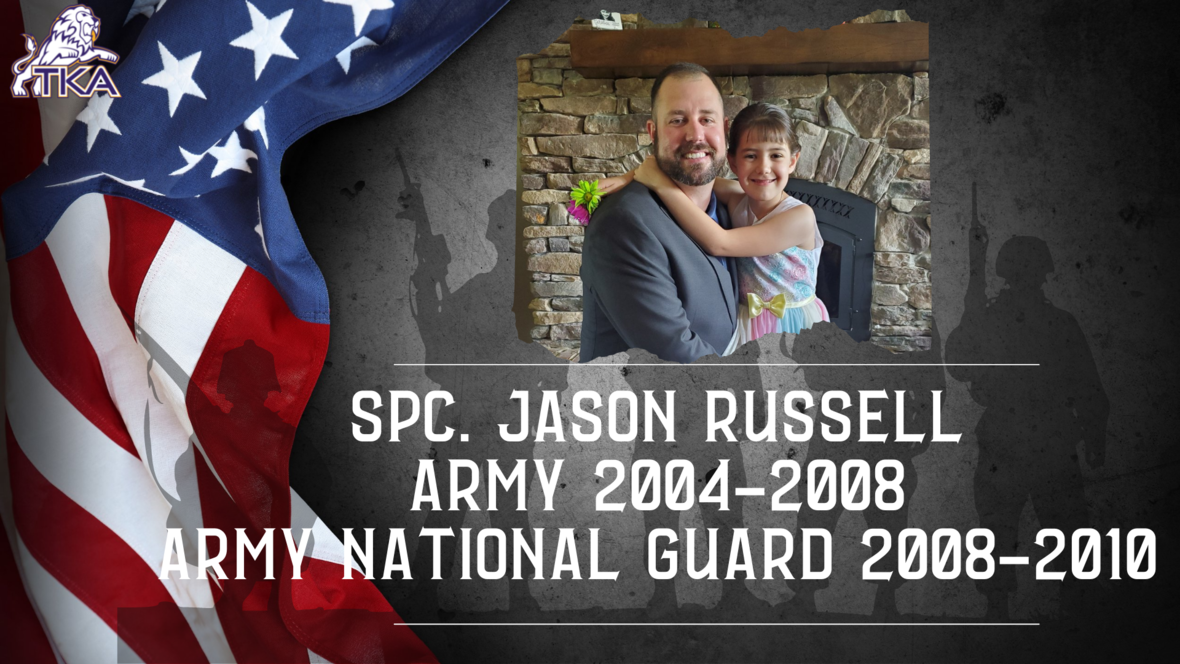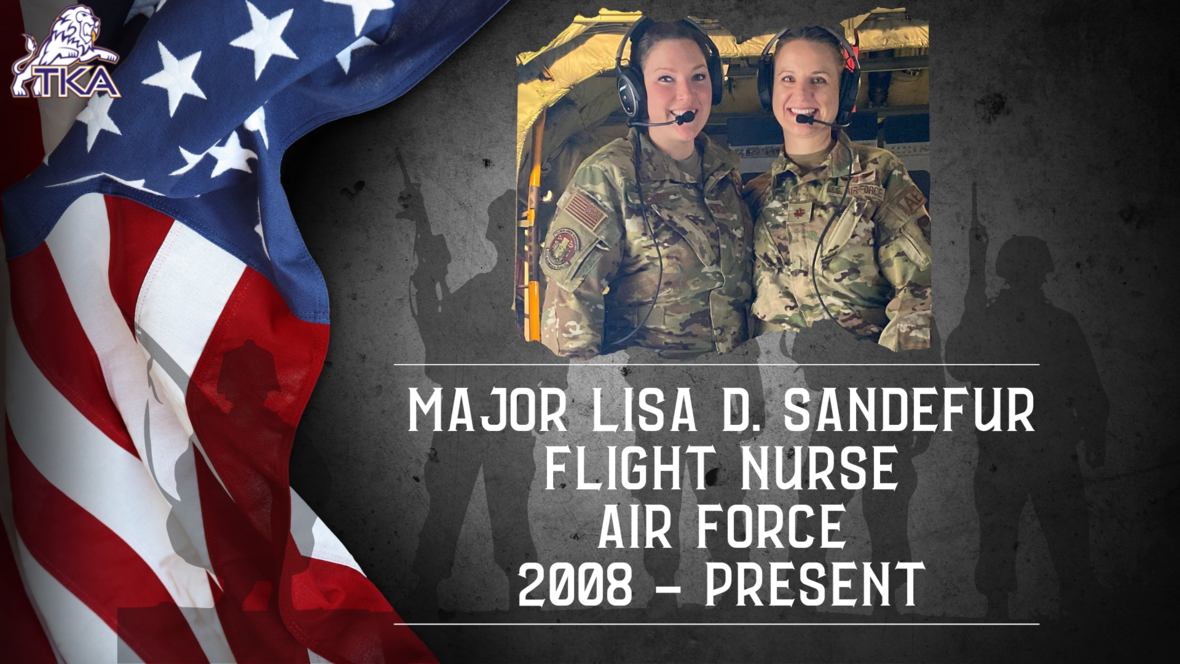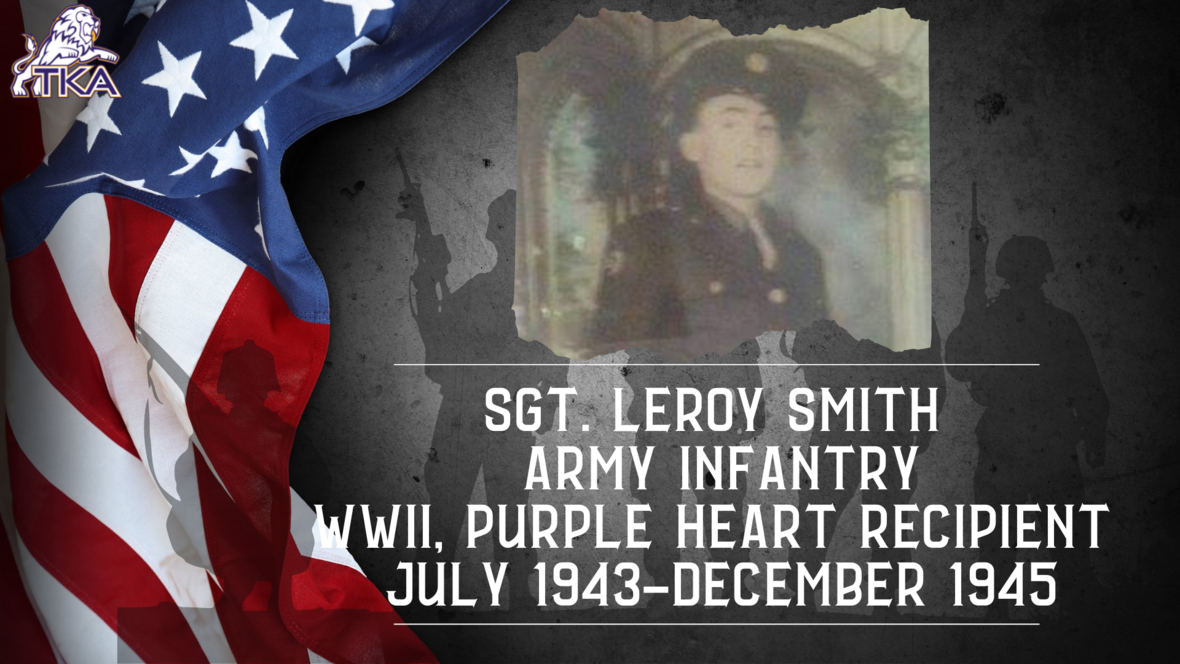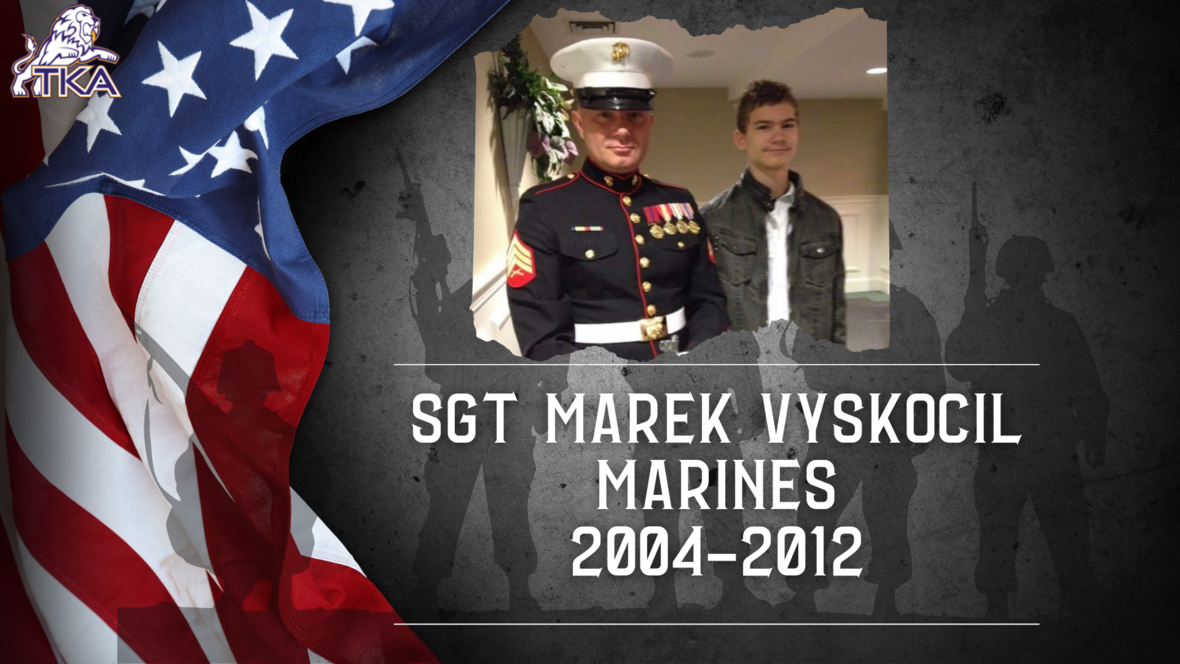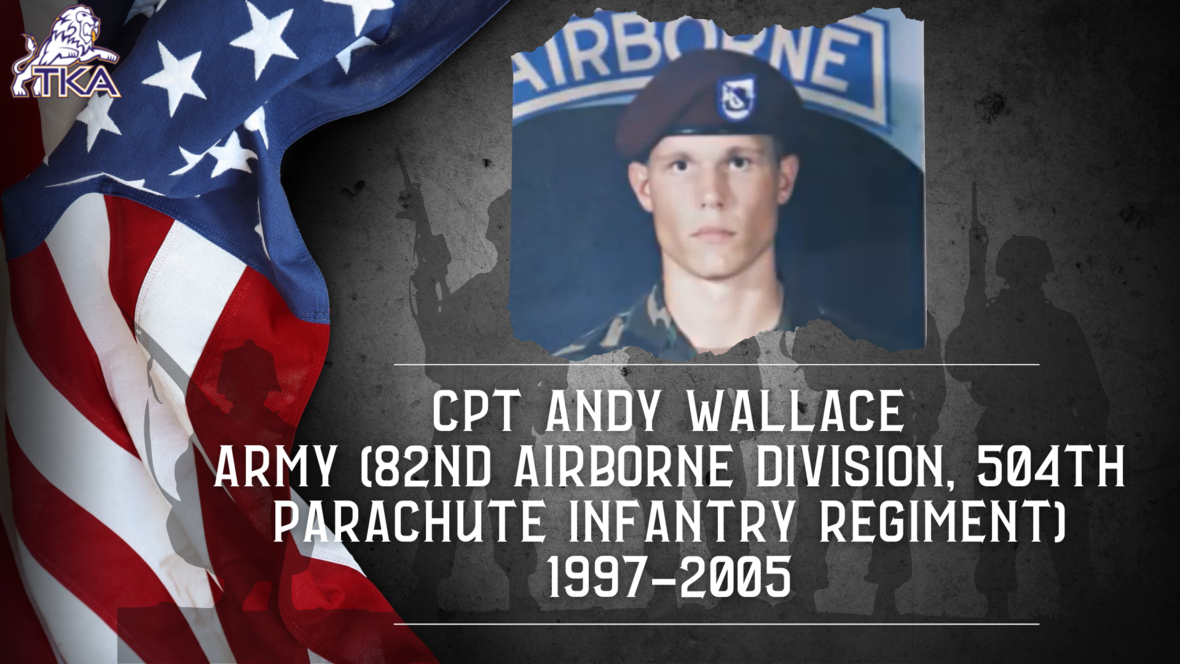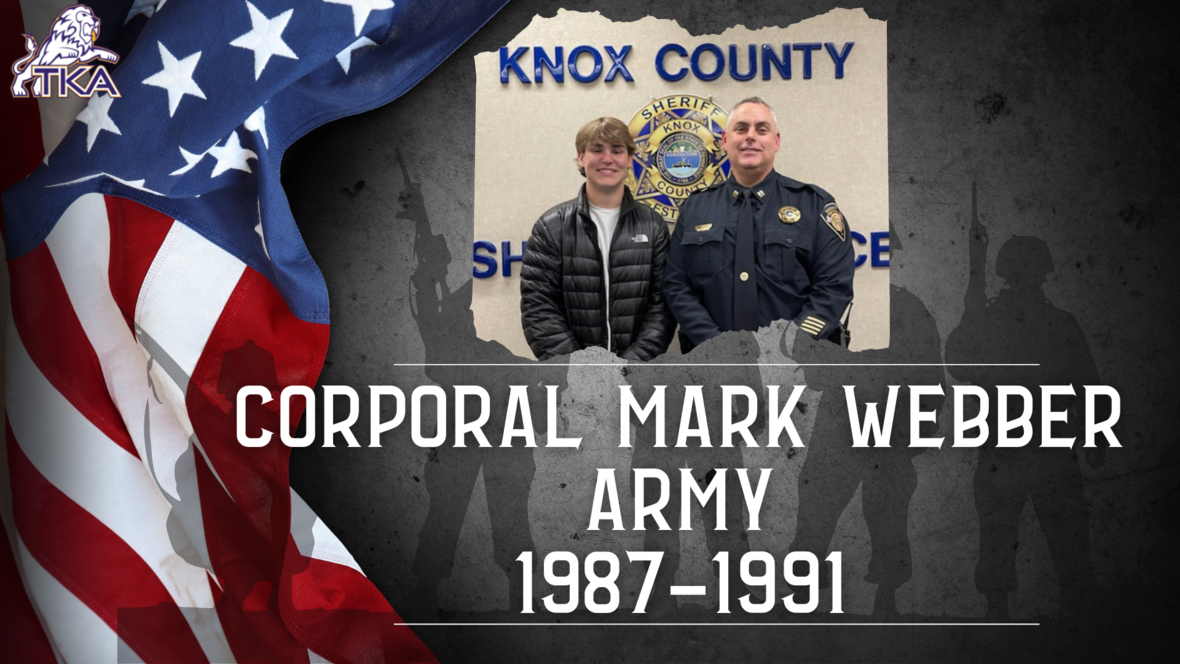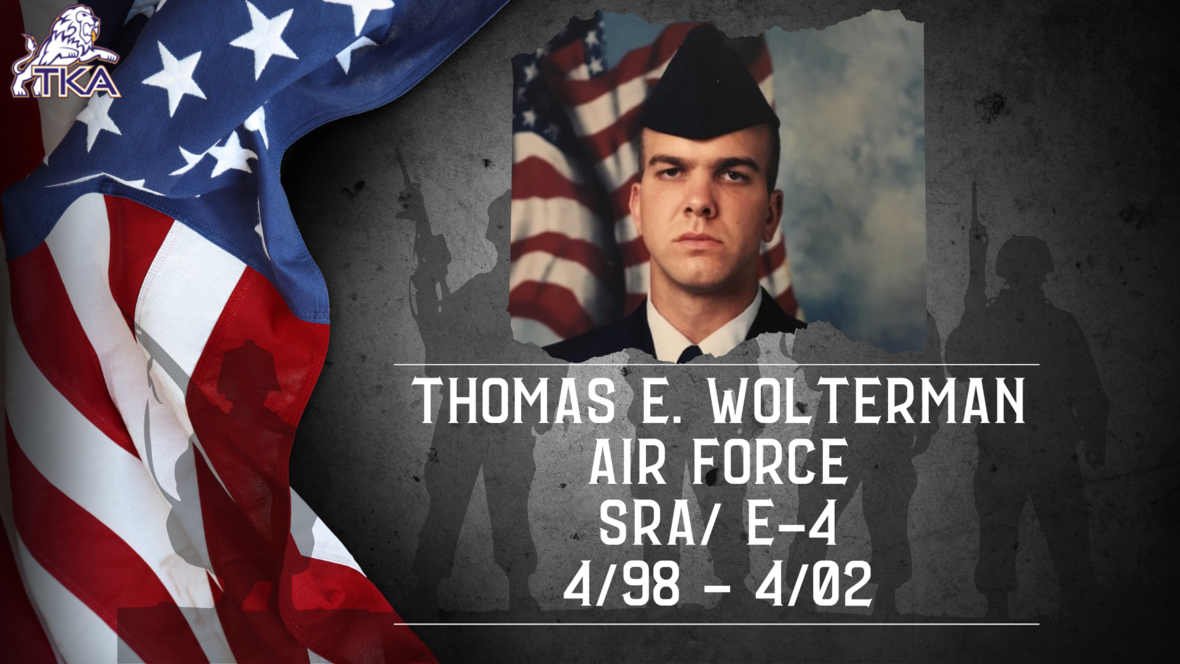table div table+table+table+table+table+table+table+table+table+table+table+table+table+table+table+table+table+table+table+table+table+table+table+table+table+table+table+table+table+table+table+table+table+table+table+table+table div table{width:100%;padding:0}table div table+table+table+table+table+table+table+table+table+table+table+table+table+table+table+table+table+table+table+table+table+table+table+table+table+table+table+table+table+table+table+table+table+table+table+table+table div table img{width:96.23%;padding:0;float:none}table div table+table+table+table+table+table+table+table+table+table+table+table+table+table+table+table+table+table+table+table+table+table+table+table+table+table+table+table+table+table+table+table+table+table+table+table+table div table td{width:100%;padding:0 1.88% 18px}/* styles */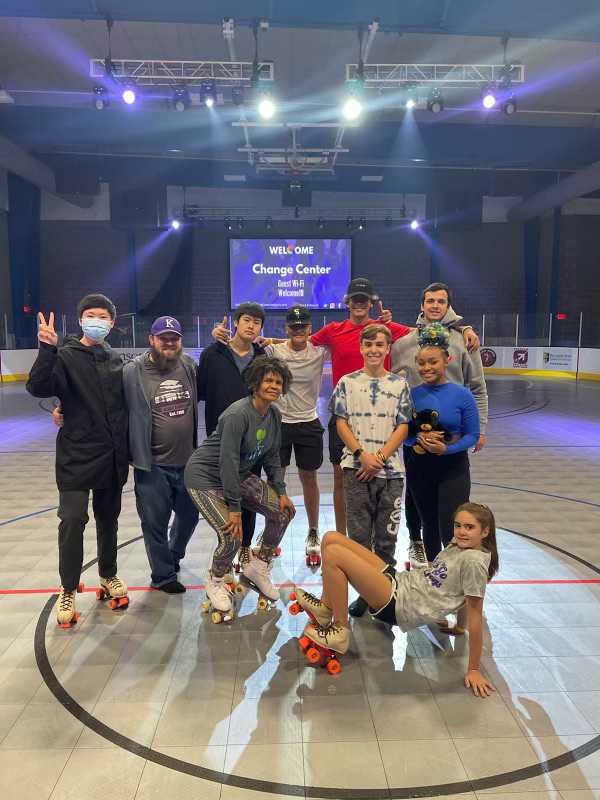# Residence Life in Action

This weekend, our residence life students went to the Change Center in Knoxville. They were able to skate, eat, and spend time together!

 table div table+table+table+table+table+table+table+table+table+table+table+table+table+table+table+table+table+table+table+table+table+table+table+table+table+table+table+table+table+table+table+table+table+table+table+table+table+table+table div table{width:100%;padding:0}table div table+table+table+table+table+table+table+table+table+table+table+table+table+table+table+table+table+table+table+table+table+table+table+table+table+table+table+table+table+table+table+table+table+table+table+table+table+table+table div table img{width:96.23%;padding:0;float:none}table div table+table+table+table+table+table+table+table+table+table+table+table+table+table+table+table+table+table+table+table+table+table+table+table+table+table+table+table+table+table+table+table+table+table+table+table+table+table+table div table td{width:100%;padding:0 1.88% 18px}/* styles */table div table+table+table+table+table+table+table+table+table+table+table+table+table+table+table+table+table+table+table+table+table+table+table+table+table+table+table+table+table+table+table+table+table+table+table+table+table+table+table+table+table div table{width:100%;padding:0}table div table+table+table+table+table+table+table+table+table+table+table+table+table+table+table+table+table+table+table+table+table+table+table+table+table+table+table+table+table+table+table+table+table+table+table+table+table+table+table+table+table div table img{width:96.23%;padding:0;float:none}table div table+table+table+table+table+table+table+table+table+table+table+table+table+table+table+table+table+table+table+table+table+table+table+table+table+table+table+table+table+table+table+table+table+table+table+table+table+table+table+table+table div table td{width:100%;padding:0 1.88% 18px}/* styles */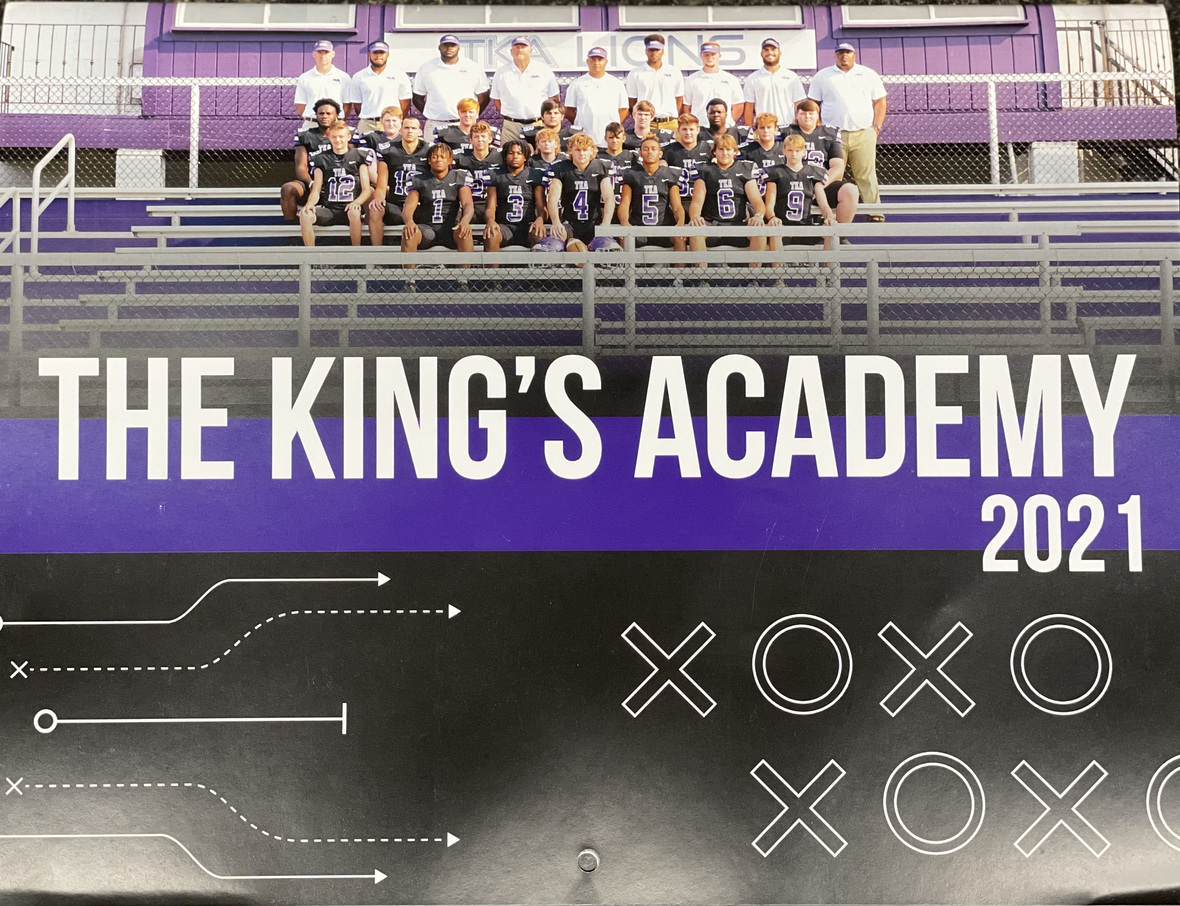# 2021-2022 Football Calendar

This year's football calendars are for sale for \$10! Stop by the school office to pick up your copy today!

 table div table+table+table+table+table+table+table+table+table+table+table+table+table+table+table+table+table+table+table+table+table+table+table+table+table+table+table+table+table+table+table+table+table+table+table+table+table+table+table+table+table+table+table div table{width:100%;padding:0}table div table+table+table+table+table+table+table+table+table+table+table+table+table+table+table+table+table+table+table+table+table+table+table+table+table+table+table+table+table+table+table+table+table+table+table+table+table+table+table+table+table+table+table div table img{width:96.23%;padding:0;float:none}table div table+table+table+table+table+table+table+table+table+table+table+table+table+table+table+table+table+table+table+table+table+table+table+table+table+table+table+table+table+table+table+table+table+table+table+table+table+table+table+table+table+table+table div table td{width:100%;padding:0 1.88% 18px}/* styles */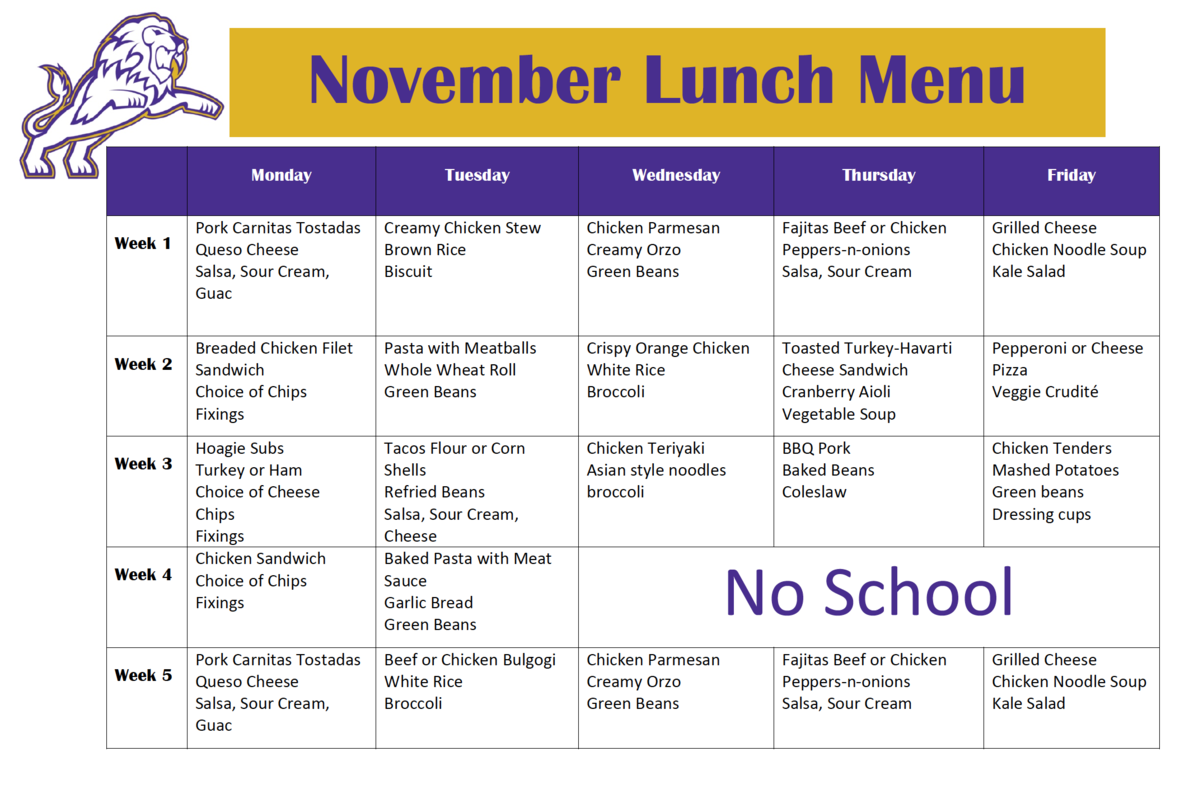table div table+table+table+table+table+table+table+table+table+table+table+table+table+table+table+table+table+table+table+table+table+table+table+table+table+table+table+table+table+table+table+table+table+table+table+table+table+table+table+table+table+table+table+table+table div table{width:100%;padding:0}table div table+table+table+table+table+table+table+table+table+table+table+table+table+table+table+table+table+table+table+table+table+table+table+table+table+table+table+table+table+table+table+table+table+table+table+table+table+table+table+table+table+table+table+table+table div table img{width:96.23%;padding:0;float:none}table div table+table+table+table+table+table+table+table+table+table+table+table+table+table+table+table+table+table+table+table+table+table+table+table+table+table+table+table+table+table+table+table+table+table+table+table+table+table+table+table+table+table+table+table+table div table td{width:100%;padding:0 1.88% 18px}/* styles */# Covid-19 Procedures

While we are excited to offer in-person education, we must maintain a safe learning environment for all students, faculty/staff, and additional stakeholders.

To do so, we will be working closely with the Sevier County Health Department regarding Covid-19 protocols.

 table div table+table+table+table+table+table+table+table+table+table+table+table+table+table+table+table+table+table+table+table+table+table+table+table+table+table+table+table+table+table+table+table+table+table+table+table+table+table+table+table+table+table+table+table+table+table+table div table{width:100%;padding:0}table div table+table+table+table+table+table+table+table+table+table+table+table+table+table+table+table+table+table+table+table+table+table+table+table+table+table+table+table+table+table+table+table+table+table+table+table+table+table+table+table+table+table+table+table+table+table+table div table img{width:96.23%;padding:0;float:none}table div table+table+table+table+table+table+table+table+table+table+table+table+table+table+table+table+table+table+table+table+table+table+table+table+table+table+table+table+table+table+table+table+table+table+table+table+table+table+table+table+table+table+table+table+table+table+table div table td{width:100%;padding:0 1.88% 18px}/* styles */table div table+table+table+table+table+table+table+table+table+table+table+table+table+table+table+table+table+table+table+table+table+table+table+table+table+table+table+table+table+table+table+table+table+table+table+table+table+table+table+table+table+table+table+table+table+table+table+table+table div table{width:100%;padding:0}table div table+table+table+table+table+table+table+table+table+table+table+table+table+table+table+table+table+table+table+table+table+table+table+table+table+table+table+table+table+table+table+table+table+table+table+table+table+table+table+table+table+table+table+table+table+table+table+table+table div table img{width:96.23%;padding:0;float:none}table div table+table+table+table+table+table+table+table+table+table+table+table+table+table+table+table+table+table+table+table+table+table+table+table+table+table+table+table+table+table+table+table+table+table+table+table+table+table+table+table+table+table+table+table+table+table+table+table+table div table td{width:100%;padding:0 1.88% 18px}/* styles */